# OOF: Finite Element Analysis of Microstructures

valeriaNext: Graphics Drawers Up: Element Types and their Previous: zimmer and zimmer2   Contents

Subsections

## valeria

This element was contributed by Valeria Cannillo. It is designed to fail (i.e. mutate) following the Weibull law: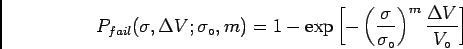(4.2)

that correlates the probability of failure to the stress state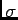in a volume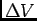and to two Weibull parameters (a shape factor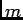and a scale parameter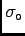).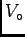is the reference volume - the specimen volume for which the Weibull parameters were determined.

A failure probability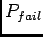is computed in every element from Eq. (4.2), using the maximum principal stress as. Each element has an area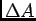that is used to determine the volumein Eq. (4.2) through the characteristic element thickness.

Each element is then randomly assigned a test probability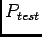from a uniform distribution in the interval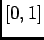. The element fractures if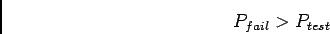(4.3)

The process of mutation is similar to that for the damage element (Section 4.5.10):

1. if Eq. (4.3) is favorable for cracking, the direction of the maximum principle stress is found

2. Modification of the stiffness matrix proceeds as in the damage element

### Parameters

orientation
As with the damage element. this is meaningless.

thermoelastic coefficients
All thermoelastic coefficients for a undamaged element are isotropic.

weibull_m
The Weibull parameter. [dimensionless]

weibull_s0
The Weibull parameter. [stress]

weibull_a0
The reference area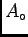(related to the reference volumethrough the characteristic element thickness) [area]

knockdown1
The factor which multiplies the rotated stiffness matrix's Czzz z component during a mutation. This should be a small number, if the element is really to be cracked''. [dimensionless]

knockdown2
The factor which multiplies the rotated stiffness matrix's remaining Cijkl components for at least one of ijkl=z during a mutation. [dimensionless]

only_once
If true, an element which has been damaged during one mutate command will not be damaged further on subsequent mutate commands. If false, the knockdown factors may be applied multiple times. Default: falseNext: Graphics Drawers Up: Element Types and their Previous: zimmer and zimmer2   Contents
/* Send mail to the OOF Team *//* Go to the OOF Home Page */